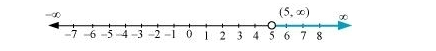# Solve the following inequalities and represent the solution graphically on number line:

Question:

Solve the following inequalities and represent the solution graphically on number line:

$3 x-7>2(x-6), 6-x>11-2 x$

Solution:

$3 x-7>2(x-6)$

$\Rightarrow 3 x-7>2 x-12$

$\Rightarrow 3 x-2 x>-12+7$

$\Rightarrow x>-5 \ldots(1)$

$6-x>11-2 x$

$\Rightarrow-x+2 x>11-6$

$\Rightarrow x>5 \ldots(2)$

From (1) and (2), it can be concluded that the solution set for the given system of inequalities is. The solution of the given system of inequalities can be represented on number line as